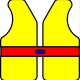## Converting metres & centimetres, length, area and volume

Many people incorrectly assume that as there are 100 cm in a metre then there must also be 100 cm2 in 1 m2 and 100 cm3 and 1 m3. Working through the pictures below should convince you that there are:

100 cm in 1 m but
10,000 cm2 in 1 m2
and 1,000,000 cm3 in 1 m3

### Length1 m = 100 cm### Area1 m2    = 100 × 100 = 10 000 cm2

= 104 cm2### Volume1 m3    =  100 × 100 × 100 = 1 000 000 cm3

= 106 cm3one litre = 1000 cm3

1 l = 1000 cm3

1 m3 = 1000 l

Now read this post in conjunction with the one on units for area and volume! The units for area are square metres and the units for volume are cubic metres. Don’t confuse either of these with metres square or metres cubed!## Difference between Square Metres and Metres Squared

### Updated 04/01/2020 (see below)Metre is the unit of length in the SI system and square metres is the SI units for calculating area.  The confusion arises when we see metres squared written or spoken. People cannot make out the difference between square metres and metres squared and assume they are the same, which they are not!

For example

If a square room has a length of 2 metres and is 2 metres in breadth, you can easily calculate its area with this formula.

 Area= Length x Breadth A=l × b 2 metres x 2 metres A = 2 m × 2 m 4 square metres A = 4 m2

The room has an area of 4 square metres

If you say that this is 4 metres squared what you mean is an area which has the length of 4 metres and you are multiplying it by a breadth of 4 metres which would give you an area of 16 square metres and not 4 square metres. That gives you a very different area.

 An Area = 4 metres squared 4 metres x 4 metres 16 square metres

So if someone asks you the correct area of the room mentioned above, you should say that the area is 4 square metres both of which are correct answers.

But beware more confusion arises as 1 m x 1 m= 1 square metres while 1 metre squared is also the same size as 1 × 1 = 1. You just get there by different routes.

Even though the unit looks like it is written as metres squared you pronounce it square metres.

Hope this clears any confusion you might have on this one!

Actually I ought to put a post script in!

The same applies to volumes
The correct SI unit for volume is cubic metres, (or in Chemistry they might use cubic centimetres). If you say metres cubed you mean that this is the length of one side and you need to cube this value to get the volume.This cube could be described as 125 cubic centimetres or 5 centimetres cubed.

I wasn’t sure that I ought to have posted this, but it looks like it is less well understood than I imagined, definitely my only popular post!Thanks to Andy and Gareth Lewis Maths tuition for these additional thoughts.

Hi, the examples that you have given for metre square and square metre are incorrect.
2 metre square = 4 square metre (2×2=4)
2 square metre = 1 metre x 2 metre (1×2=2)

Andy

XxxxxxxxxxxxxxxxxxxxxxxxX

Good article. Andy’s alternative examples are also correct.

As well as the difference in size between square metres and metres squared (except when you have zero of each or one of each) there is a difference in shape. A metre square is a square with sides one metre in length – it refers to the shape and the side length, not the area. By contrast, a square metre is an area and can be any shape. A square metre could, for example, be in the shape of an oblong of dimensions 50cm x 2m, or in the shape of an A0 sheet, or 16 A4 sheets in any pattern.

After all the comments on here I decided to contact the people who know this stuff as metrology  is their business.

So I contacted the NPL (National Physical Laboratory, this is

Andrew Hanson, MBE BSc (Hons) CPhys, Outreach Manager, National Physical Laboratory, Hampton Rd | Teddington | Middlesex | UK | TW11 0LW

From P162 of the SI Brochure (most recent – 9th edition 2019), the area unit definition is:

NAME: square metre

SYMBOL: m2

Almost certainly unchanged from when you first wrote your blog item.

As an example from the definition, spoken ‘9 square metres’, written ‘9 m2‘ are both 9 times the area of the unitary square metre.

The confusion arises from reading m2 as the words ‘metres squared’. These words are NOT defined in the SI system. So with no formal meaning, ‘9 metres squared’ could indeed be taken to mean 9×9 m2 or 9 m2 – there is no definitive statement one way or the other.

I think that m2 should be said out loud as ‘square metres’, so don’t take your blog item down, by all means say I agree.

Actually, if you just do the right/formal thing – use the words ‘square metre’ out loud and write as m2, and never, never even whisper ‘metre squared’, there is NO CONFUSION.

A formal way to do describe things with well defined, precise, scientific language avoids the types of problem you describe.

However, common parlance is commonly improperly used to describe scientific parameters, and these words without clear meaning cause confusion.

Most people don’t discriminate – though metrologists, and people who set and mark exam papers take care to get it right.

I am sure people passionate about English who know dictionary meaning of words are similarly riled by common misuse.